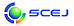\$B9V1i%W%m%0%i%`!J%;%C%7%g%sJL!K(B

English page

\$BH?1~9)3X(B

D214-D219

\$B:G=*99?7F|;~!'(B2019-12-10 12:54:57
\$B9V1i(B
\$B;~9o(B
\$B9V1i(B
\$BHV9f(B
\$B9V1iBjL\!?H/I=\$B%-!<%o!<%I(B\$BJ,N`(B
\$BHV9f(B
\$B\$BHV9f(B
D \$B2q>l!&Bh(B 2 \$BF|(B
(13:20\$B!A(B15:20) (\$B:BD9(B \$BEBB<(B \$B=\$!&HSB<(B \$B7r
13:20\$B!A(B 13:40D214\$B%Y%s%<%s\$+\$i\$NG.J,2rC:AG(BCVD\$BB.EY\$K?e>x5\$\$,5Z\$\\$98z2L(B
(\$B5~Bg9)(B) (\$B3X(B)\$B!{Fn(B \$BNK2O(B\$B!&(B (\$B3X(B)\$BKRLn(B \$BM%:n(B\$B!&(B (\$B3X(B)\$B>>ED(B \$BK((B\$B!&(B (\$B@5(B)\$B2O@%(B \$B85L@(B
CVD
Carbon
Steam
5-h15
13:40\$B!A(B 14:00D215\$B%h%&2=1t%a%A%k%"%s%b%K%&%`%Z%m%V%9%+%\$%HGvKl\$N?75,5\$Aj@.D9%W%m%;%93+H/(B
(\$B5~Bg9)(B) (\$B3X(B)\$B!{>>ED(B \$BK((B\$B!&(B (\$B3X(B)\$BB<>e(B \$BM@5*(B\$B!&(B (\$B3X(B)\$B>>B<(B \$BFn7n(B\$B!&(B (\$B@5(B)\$B2O@%(B \$B85L@(B
CVD
perovskite
5-h38
14:00\$B!A(B 14:20D216\$B%W%i%:%^(BCVD\$BK!\$rMQ\$\$\$F:n@=\$7\$?%7%j%+7O%,%9%P%j%"Kl\$K2C\$o\$k;DN11~NODc8:J}K!\$N8!F\$(B
(\$B5~Bg(B) (\$B3X(B)\$B!{J?ED(B \$B7,0lO/(B\$B!&(B (\$B3X(B)\$BEDCf(B \$Bg}h=0!(B\$B!&(B (\$B3X(B)\$BOF:d(B \$BCN\$B!&(B (\$B@5(B)\$B2O@%(B \$B85L@(B
gas barrier film
silica
cvd
5-h134
14:20\$B!A(B 14:40D217\$B%T%;%sM6F3BNGvKl\$K\$h\$k2D;k8w>H
(\$B?@8MBg9)(B) \$B!{9>(B \$B1'HA(B\$B!&(B \$BFbF#(B \$BfFB@(B\$B!&(B \$B<2ED(B \$B>9?N(B\$B!&(B (\$B@5(B)\$BC+20(B \$B7\$B!&(B (\$B@5(B)\$B;T66(B \$BM40l(B\$B!&(B (\$B@5(B)\$B@>;3(B \$B3P(B
Photocatalyst
Decomposition of Water
Thin Film
5-a110
14:40\$B!A(B 15:00D218\$B=
(\$B5~Bg1!9)(B) (\$B3X(B)\$B!{KL@n(B \$B>=Bg(B\$B!&(B (\$B@5(B)\$BEBB<(B \$B=\$(B\$B!&(B (\$B@5(B)\$BKR(B \$BBYJe(B\$B!&(B (\$B@5(B)\$B30NX(B \$B7r0lO:(B
packed bed reactors
design and operation
CFD
5-f63
15:00\$B!A(B 15:20D219\$B6bB0;@2=J*\$rMQ\$\$\$??eAG\$N?WB.=hM}\$K\$*\$1\$k5\$8GH?1~%b%G%k\$N9=C[(B
(\$B5~Bg9)(B) (\$B3X(B)\$B!{\$B!&(B (\$B3X(B)\$B?{C+(B \$BB@51(B\$B!&(B (\$B@5(B)\$BiCED(B \$BN40l(B\$B!&(B (\$BEl\$B!&(B \$B;3ED(B \$B97(B\$B!&(B (\$B5~Bg9)(B) (\$B@5(B)\$B2O@%(B \$B85L@(B
Gas-solid reaction
Metal oxide
Hydrogen removal
5-i51

\$B9V1iH/I=%W%m%0%i%`(B
\$B2=3X9)3X2q(B \$BI1O)Bg2q(B2019(C) 2019 \$B8x1W
Most recent update: 2019-12-10 12:54:57
E-mail: inquiry-ks2019www3.scej.org
This page was generated byeasp 2.44; proghtml 2.42 (C)1999-2018 kawase